# Rename specific column(s) in Pandas

Given a pandas Dataframe, let’s see how to rename specific column(s) names using various methods.

First, let’s create a Dataframe:

## Python3

 `# import pandas package ` `import` `pandas as pd ` ` `  `# defining a dictionary ` `d ``=` `{``"Name"``: [``"John"``, ``"Mary"``, ``"Helen"``], ` `     ``"Marks"``: [``95``, ``75``, ``99``], ` `     ``"Roll No"``: [``12``, ``21``, ``9``]} ` ` `  `# creating the pandas data frame ` `df ``=` `pd.DataFrame(d) ` ` `  `df`

Output:Method 1: Using Dataframe.rename().

This method is a way to rename the required columns in Pandas. It allows us to specify the columns’ names to be changed in the form of a dictionary with the keys and values as the current and new names of the respective columns.

Example 1: Renaming a single column.

## Python3

 `# import pandas package ` `import` `pandas as pd ` ` `  `# defining a dictionary ` `d ``=` `{``"Name"``: [``"John"``, ``"Mary"``, ``"Helen"``], ` `     ``"Marks"``: [``95``, ``75``, ``99``], ` `     ``"Roll No"``: [``12``, ``21``, ``9``]} ` ` `  `# creating the pandas data frame ` `df ``=` `pd.DataFrame(d) ` ` `  `# displaying the columns  ` `# before renaming ` `print``(df.columns) ` ` `  `# renaming the column "A" ` `df.rename(columns ``=` `{``"Name"``: ``"Names"``},  ` `          ``inplace ``=` `True``) ` ` `  `# displaying the columns after renaming ` `print``(df.columns)`

Output: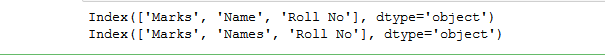Example 2: Renaming multiple columns.

## Python3

 `# import pandas package ` `import` `pandas as pd ` ` `  `# defining a dictionary ` `d ``=` `{``"Name"``: [``"John"``, ``"Mary"``, ``"Helen"``], ` `     ``"Marks"``: [``95``, ``75``, ``99``], ` `     ``"Roll No"``: [``12``, ``21``, ``9``]} ` ` `  `# creating the pandas dataframe ` `df ``=` `pd.DataFrame(d) ` ` `  `# displaying the columns before renaming ` `print``(df.columns) ` ` `  `# renaming the columns ` `df.rename({``"Name"``: ``"Student Name"``,  ` `           ``"Marks"``: ``"Marks Obtained"``,  ` `           ``"Roll No"``: ``"Roll Number"``},  ` `          ``axis ``=` `"columns"``, inplace ``=` `True``) ` ` `  `# displaying the columns after renaming ` `print``(df.columns) `

Output: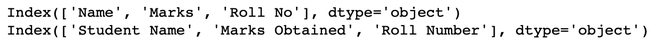Example 3: Passing the lambda function to rename columns.

## Python3

 `# using the same modified dataframe ` `# df from Renaming Multiple Columns ` `# this adds ':' at the end  ` `# of each column name ` `df ``=` `df.rename(columns ``=` `lambda` `x: x``+``':'``) ` ` `  `# printing the columns ` `print``(df.columns) `

Output: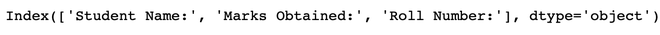The lambda function is a small anonymous function that can take any number of arguments but can only have one expression. We can use it if we have to modify all columns at once. It is useful if the number of columns is large, and it is not an easy task to rename them using a list or a dictionary (a lot of code, phew!). In the above example, we used the lambda function to add a colon (‘:’) at the end of each column name.

Method 2: Using the values attribute.

We can use values attribute on the column we want to rename and directly change it.

## Python3

 `# using the same modified dataframe  ` `# df from Renaming Multiple Columns ` `# Renaming the third column ` `df.columns.values[``2``] ``=` `"Roll Number"`  ` `  `# printing the columns ` `print``(df.columns) `

Output: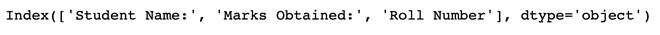Method 3: Using a new list of column names.

We pass the updated column names as a list to rename the columns. The length of the list we provide should be the same as the number of columns in the data frame. Otherwise, an error occurs.

## Python3

 `# Creating a list of new columns ` `df_cols ``=` `[``"Student Name"``,  ` `           ``"Marks Obtained"``, ` `           ``"Roll Number"``] ` ` `  `# printing the columns  ` `# before renaming ` `print``(df.columns) ` ` `  `# Renaming the columns ` `df.columns ``=` `df_cols ` ` `  `# printing the columns  ` `# after renaming ` `print``(df.columns) `

Output: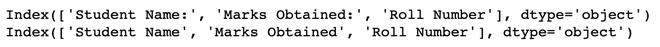Method 4: Using the Dataframe.columns.str.replace().

In general, if the number of columns in the Pandas dataframe is huge, say nearly 100, and we want to replace the space in all the column names (if it exists) by an underscore. It is not easy to provide a list or dictionary to rename all the columns. Therefore, we use a method as below –

## Python3

 `# printing the column  ` `# names before renaming ` `print``(df.columns) ` ` `  `# Replacing the space in column  ` `# names by an underscore ` `df.columns ``=` `df.columns.``str``.replace(``' '``, ``'_'``) ` ` `  `# printing the column names  ` `# after renaming ` `print``(df.columns) `

Output: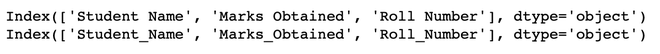Also, other string methods such as str.lower can be used to make all the column names lowercase.

Note: Suppose that a column name is not present in the original data frame, but is in the dictionary provided to rename the columns. By default, the errors parameter of the rename() function has the value ‘ignore.’ Therefore, no error is displayed and, the existing columns are renamed as instructed. In contrast, if we set the errors parameter to ‘raise,’ then an error is raised, stating that the particular column does not exist in the original data frame.

Below is an example of the same:

Example 1: No error is raised as by default errors is set to ‘ignore.’

## Python3

 `# NO ERROR IS RAISED  ` ` `  `# import pandas package ` `import` `pandas as pd ` ` `  `# defining a dictionary ` `d ``=` `{``"A"``: [``1``, ``2``, ``3``],  ` `     ``"B"``: [``4``, ``5``, ``6``]} ` ` `  `# creating the pandas dataframe ` `df ``=` `pd.DataFrame(d) ` ` `  `# displaying the columns before renaming ` `print``(df.columns) ` ` `  `# renaming the columns ` `# column "C" is not in  ` `# the original dataframe  ` `# errors parameter is ` `# set to 'ignore' by default ` `df.rename(columns ``=` `{``"A"``: ``"a"``, ``"B"``: ``"b"``, ` `                     ``"C"``: ``"c"``},  ` `          ``inplace ``=` `True``) ` ` `  `# displaying the columns ` `# after renaming ` `print``(df.columns) `

Output: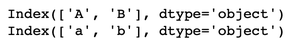Example 2: Setting the parameter errors to ‘raise.’ Error is raised ( column C does not exist in the original data frame.)

## Python3

 `# ERROR IS RAISED  ` ` `  `# import pandas package ` `import` `pandas as pd ` ` `  `# defining a dictionary ` `d ``=` `{``"A"``: [``1``, ``2``, ``3``], ` `     ``"B"``: [``4``, ``5``, ``6``]} ` ` `  `# creating the pandas dataframe ` `df ``=` `pd.DataFrame(d) ` ` `  `# displaying the columns ` `# before renaming ` `print``(df.columns) ` ` `  `# renaming the columns ` `# column "C" is not in the  ` `# original dataframe setting ` `# the errors parameter to 'raise' ` `df.rename(columns ``=` `{``"A"``: ``"a"``, ``"B"``: ``"b"``,  ` `                     ``"C"``: ``"c"``},  ` `          ``inplace ``=` `True``, errors ``=` `'raise'``) ` ` `  `# displaying the columns  ` `# after renaming ` `print``(df.columns) `

Output: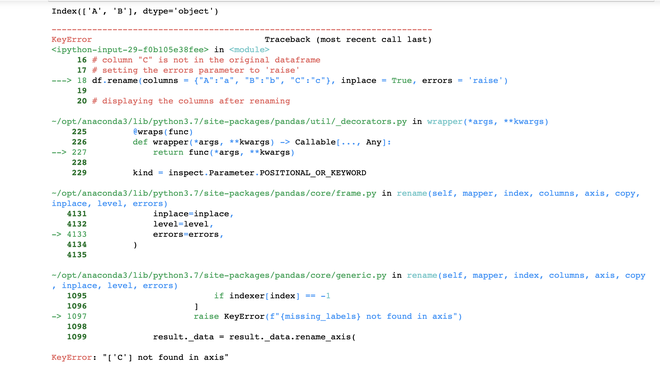Error is raised

Whether you're preparing for your first job interview or aiming to upskill in this ever-evolving tech landscape, GeeksforGeeks Courses are your key to success. We provide top-quality content at affordable prices, all geared towards accelerating your growth in a time-bound manner. Join the millions we've already empowered, and we're here to do the same for you. Don't miss out - check it out now!

Previous
Next